# Calculations in crosstabs

If we create at a crosstab based on Profit per Customer Country (attribute) and by Time Year (from the Sales cube), we should end up with a crosstab with 14 rows and 4 columns (not counting the Total row/column) like this: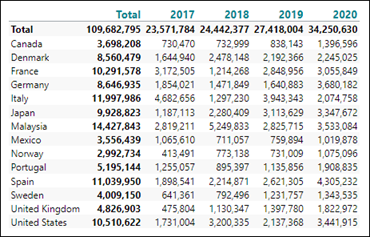This crosstab, with the reference synatx in mind, can be broken down to:

• Columns: Years, d1 to d4 – or d-1 to d-4 (if counted from right to left).
• Rows: Countries, d1 to d14 – or d-1 to d-14 (if counted from bottom to top).
• Measures: Profit, m1. This crosstab contains only one measure.

To calculate the difference between e.g. the last two years, we can do this with a Custom calculation: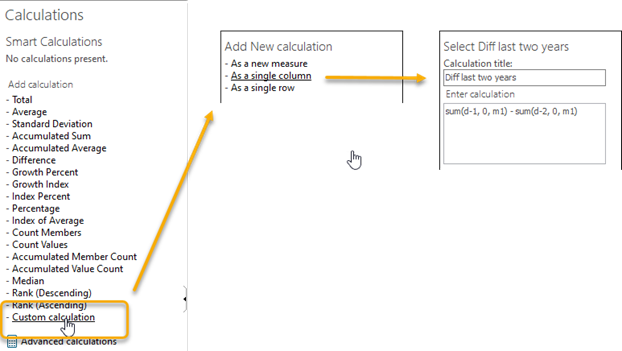We could also add an extra calculated column, Growth last two years, like this: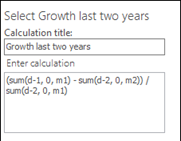With Growth number formatted as Percent we should end up with a result like this: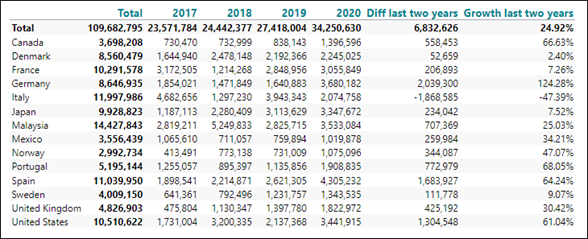Furthermore, we could add an average calculation for each year like this: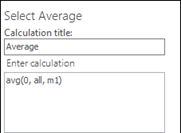With the average calculation included, we should get a result like this: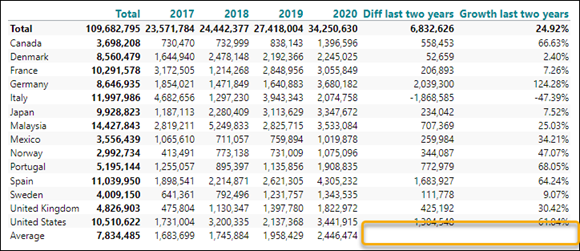Notice the two blank cells in the intersection between the calculated columns and the calculated row. They are blank simply because TARGIT does not know if you prefer to calculate an average for the diff and growth valuesor if you prefer to calculate a diff and a growth for the average values.

Supposing we decide to calculate the diff and the growth for the average values, we should re-open the the Diff and the Growth calculation to make sure that the Average is included in the Calculate in intersection setting: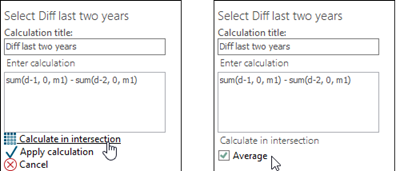In this way, we will eventually get to a result like this: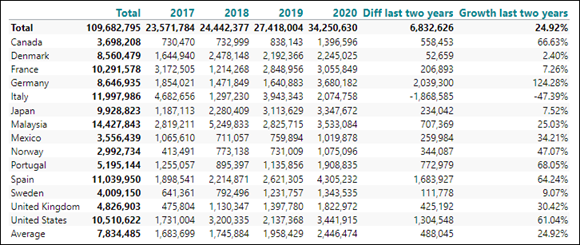0 out of 0 found this helpful

• How do I change the order of the columns? I need a calculated field between 2 measures.

• Hi Jora,

For calculations that have been added as 'Single column' or 'Single row', you can just drag and drop the calculation into its proper place.

For calculations that have been added 'As a new measure', the calculated measure will always come after the original measures. If you need such a calculated measure to come between two original measures, you will have to follow this approach:

1. Create your crosstab with the two original measures.
2. Add your calculation 'As a new measure'. (It will be placed after the two original measures.)
3. Copy your original second measure by adding another calculation 'As a new measure'. The syntax would probably be something like: sum(0, 0, m2).
4. From the Properties tab, Visibility, hide your original second measure.

In this way it will appear as if the new calculated measure is between the two original measures.

Best regards,

Ole

• Thank you!